# Geiger–Marsden experiment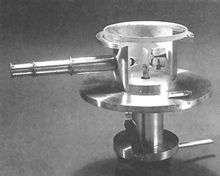A replica of one of Geiger and Marsden's apparatus

The Geiger–Marsden experiment(s) (also called the Rutherford gold foil experiment) were a landmark series of experiments by which scientists discovered that every atom contains a nucleus where its positive charge and most of its mass are concentrated. They deduced this by measuring how an alpha particle beam is scattered when it strikes a thin metal foil. The experiments were performed between 1908 and 1913 by Hans Geiger and Ernest Marsden under the direction of Ernest Rutherford at the Physical Laboratories of the University of Manchester.

## Summary

### Contemporary theories of atomic structure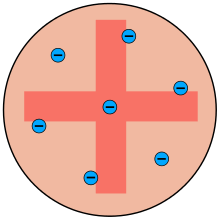The popular theory of atomic structure at the time of Rutherford's experiment was the "plum pudding model". This model was devised by Lord Kelvin and further developed by J. J. Thomson. Thomson was the scientist who discovered the electron, and that it was a component of every atom. Thomson believed the atom was a sphere of positive charge throughout which the electrons were distributed, a bit like plums in a Christmas pudding. The existence of protons and neutrons was unknown at this time. They knew atoms were very tiny (Rutherford assumed they were in the order of 10−8 m in radius). This model was based entirely on classical (Newtonian) physics; the current accepted model uses quantum mechanics.

Thomson's model was not universally accepted even before Rutherford's experiments. Thomson himself was never able to develop a complete and stable model of his concept. A Japanese scientist named Hantaro Nagaoka rejected Thomson's model on the grounds that opposing charges cannot penetrate each other. He proposed instead that electrons orbit the positive charge like the rings around Saturn.

### Implications of the plum pudding model

An alpha particle is a sub-microscopic, positively charged particle of matter. According to Thomson's model, if an alpha particle were to collide with an atom, it would just fly straight through, its path being deflected by at most a fraction of a degree. At the atomic scale, the concept of "solid matter" is meaningless, so the alpha particle would not bounce off the atom like a marble; it would be affected only by the atom's electric fields, and Thomson's model predicted that the electric fields in an atom are just too weak to affect a passing alpha particle much (alpha particles tend to move very fast). Both the negative and positive charges within the Thomson atom are spread out over the atom's entire volume. According to Coulomb's Law, the less concentrated a sphere of electric charge is, the weaker its electric field at its surface will be.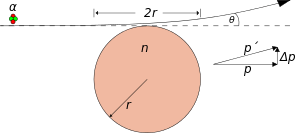As a worked example, consider an alpha particle passing tangentially to a Thomson gold atom, where it will experience the electric field at its strongest and thus experience the maximum deflection θ. Since the electrons are very light compared to the alpha particle, their influence can be neglected and the atom can be seen as a heavy sphere of positive charge.

Qn = positive charge of gold atom = 79 e = 1.266×10−17 C
Qα = charge of alpha particle = 2 e = 3.204×10−19 C
r = radius of a gold atom = 1.44×10−10 m
vα = velocity of alpha particle = 1.53×107 m/s
mα = mass of alpha particle = 6.645×10−27 kg
k = Coulomb's constant = 8.998×109 N·m2/C2

Using classical physics, the alpha particle's lateral change in momentum Δp can be approximated using the impulse of force relationship and the Coulomb force expression:The above calculation is but an approximation of what happens when an alpha particle comes near a Thomson atom, but it is clear that the deflection at most will be in the order of a small fraction of a degree. If the alpha particle were to pass through a gold foil some 400 atoms thick and experience maximal deflection in the same direction (unlikely), it would still be a small deflection.

### The outcome of the experiments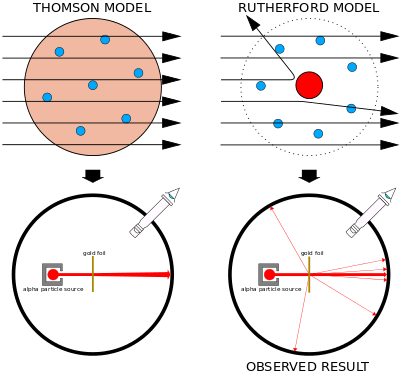Left: Had Thomson's model been correct, all the alpha particles should have passed through the foil with minimal scattering.
Right: What Geiger and Marsden observed was that a small fraction of the alpha particles experienced strong deflection.

At Rutherford's behest, Geiger and Marsden performed a series of experiments where they pointed a beam of alpha particles at a thin foil of metal and measured the scattering pattern by using a fluorescent screen. They spotted alpha particles bouncing off the metal foil in all directions, some right back at the source. This should have been impossible according to Thomson's model; the alpha particles should have all gone straight through. Obviously, those particles had encountered an electrostatic force far greater than Thomson's model suggested they would, which in turn implied that the atom's positive charge was concentrated in a much tinier volume than Thomson imagined.

When Geiger and Marsden shot alpha particles at their metal foils, they noticed only a tiny fraction of the alpha particles were deflected by more than 90°. Most just flew straight through the foil. This suggested that those tiny spheres of intense positive charge were separated by vast gulfs of empty space. Most particles passed through the empty space and experienced negligible deviation, while a handful struck the nuclei of the atoms and bounced right back.

Rutherford thus rejected Thomson's model of the atom, and instead proposed a model where the atom consisted of mostly empty space, with all its positive charge concentrated in its center in a very tiny volume, surrounded by a cloud of electrons.

## Timeline

### Background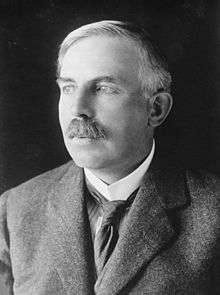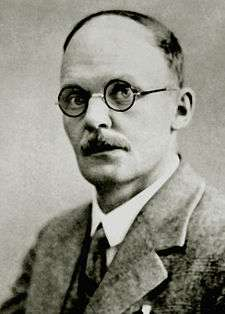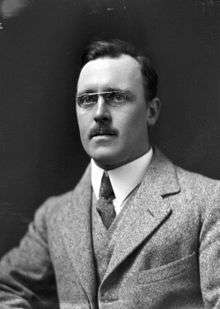Ernest Rutherford was a physics professor at the University of Manchester. He had already received numerous honors for his studies of radiation. He had discovered the existence of alpha rays, beta rays, and gamma rays, and had proved that these were the consequence of the disintegration of atoms. In 1906, he received a visit from a German physicist named Hans Geiger, and was so impressed that he asked Geiger to stay and help him with his research. Ernest Marsden was a physics undergraduate student studying under Geiger.

Alpha particles are tiny, positively charged particles that are spontaneously emitted by certain substances such as uranium and radium. Rutherford himself had discovered them in 1899. In 1908 he was trying to precisely measure their charge-to-mass ratio. To do this, he first needed to know just how many alpha particles his sample of radium was giving off (after which he would measure their total charge and divide one by the other). Alpha particles are too tiny to be seen even with a microscope, but Rutherford knew that alpha particles ionize air molecules, and if the air is within an electric field, the ions will produce an electric current. On this principle, Rutherford and Geiger designed a simple counting device which consisted of two electrodes in a glass tube. Every alpha particle that passed through the tube would create a pulse of electricity that could be counted. It was an early version of the Geiger counter.

The counter that Geiger and Rutherford built proved unreliable because the alpha particles were being too strongly deflected by their collisions with the molecules of air within the detection chamber. The highly variable trajectories of the alpha particles meant that they did not all generate the same number of ions as they passed through the gas, thus producing erratic readings. This puzzled Rutherford because he had thought that alpha particles were just too heavy to be deflected so strongly. Rutherford asked Geiger to investigate just how much matter could scatter alpha rays.

The experiments they designed involved bombarding a metal foil with alpha particles to observe how the foil scattered them in relation to their thickness and material. They used a fluorescent screen to measure the trajectories of the particles. Each impact of an alpha particle on the screen produced a tiny flash of light. Geiger worked in a darkened lab for hours on end, counting these tiny scintillations using a microscope. Rutherford lacked the endurance for this work, which is why he left it to his younger colleagues. For the metal foil, they tested a variety of metals, but they preferred gold because they could make the foil very thin, as gold is very malleable. As a source of alpha particles, Rutherford's substance of choice was radium, a substance several million times more radioactive than uranium.

### The 1908 experiment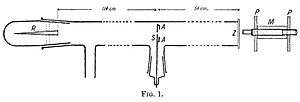This apparatus was described in a 1908 paper by Hans Geiger. It could only measure deflections of a few degrees.

A 1908 paper by Geiger, On the Scattering of α-Particles by Matter, describes the following experiment. He constructed a long glass tube, nearly two meters in length. At one end of the tube was a quantity of "radium emanation" (R) that served as a source of alpha particles. The opposite end of the tube was covered with a phosphorescent screen (Z). In the middle of the tube was a 0.9 mm-wide slit. The alpha particles from R passed through the slit and created a glowing patch of light on the screen. A microscope (M) was used to count the scintillations on the screen and measure their spread. Geiger pumped all the air out of the tube so that the alpha particles would be unobstructed, and they left a neat and tight image on the screen that corresponded to the shape of the slit. Geiger then allowed some air in the tube, and the glowing patch became more diffuse. Geiger then pumped out the air and placed some gold foil over the slit at AA. This too caused the patch of light on the screen to become more spread out. This experiment demonstrated that both air and solid matter could markedly scatter alpha particles. The apparatus, however, could only observe small angles of deflection. Rutherford wanted to know if the alpha particles were being scattered by even larger angles—perhaps larger than 90°.

### The 1909 experiment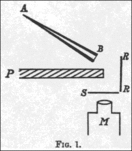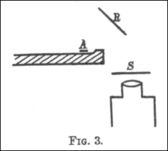In these experiments, alpha particles emitted by a radioactive source (A) were observed bouncing off a metal reflector (R) and onto a fluorescent screen (S) on the other side of a lead plate (P).

In a 1909 paper, On a Diffuse Reflection of the α-Particles, Geiger and Marsden described the experiment by which they proved that alpha particles can indeed be scattered by more than 90°. In their experiment, they prepared a small conical glass tube (AB) containing "radium emanation" (radon), "radium A" (actual radium), and "radium C" (bismuth-214); its open end sealed with mica. This was their alpha particle emitter. They then set up a lead plate (P), behind which they placed a fluorescent screen (S). The tube was held on the opposite side of plate, such that the alpha particles it emitted could not directly strike the screen. They noticed a few scintillations on the screen, because some alpha particles got around the plate by bouncing off air molecules. They then placed a metal foil (R) to the side of the lead plate. They pointed the tube at the foil to see if the alpha particles would bounce off it and strike the screen on the other side of the plate, and observed an increase in the number of scintillations on the screen. Counting the scintillations, they observed that metals with higher atomic mass, such as gold, reflected more alpha particles than lighter ones such as aluminium.

Geiger and Marsden then wanted to estimate the total number of alpha particles that were being reflected. The previous setup was unsuitable for doing this because the tube contained several radioactive substances (radium plus its decay products) and thus the alpha particles emitted had varying ranges, and because it was difficult for them to ascertain at what rate the tube was emitting alpha particles. This time, they placed a small quantity of radium C (bismuth-214) on the lead plate, which bounced off a platinum reflector (R) and onto the screen. They found that only a tiny fraction of the alpha particles that struck the reflector bounced onto the screen (in this case, 1 in 8000).

### The 1910 experiment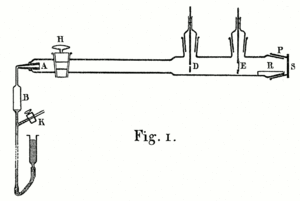This apparatus was described in 1910 paper by Geiger. It was designed to precisely measure how the scattering varied according to the substance and thickness of the foil.

A 1910 paper by Geiger, The Scattering of the α-Particles by Matter, describes an experiment by which he sought to measure how the most probable angle through which an a-particle is deflected varies with the material it passes through, the thickness of said material, and the velocity of the alpha particles. He constructed an airtight glass tube from which the air was pumped out. At one end was a bulb (B) containing "radium emanation" (radon-222). By means of mercury, the radon in B was pumped up the narrow glass pipe whose end at A was plugged with mica. At the other end of the tube was a fluorescent zinc sulfide screen (S). The microscope which he used to count the scintillations on the screen was affixed to a vertical millimeter scale with a vernier, which allowed Geiger to precisely measure where the flashes of light appeared on the screen and thus calculate the particles' angles of deflection. The alpha particles emitted from A was narrowed to a beam by a small circular hole at D. Geiger placed a metal foil in the path of the rays at D and E to observe how the zone of flashes changed. He could also vary the velocity of the alpha particles by placing extra sheets of mica or aluminium at A.

From the measurements he took, Geiger came to the following conclusions:

• the most probable angle of deflection increases with the thickness of the material
• the most probable angle of deflection is proportional to the atomic mass of the substance
• the most probable angle of deflection decreases with the velocity of the alpha particles
• the probability that a particle will be deflected by more than 90° is vanishingly small

### Rutherford mathematically models the scattering pattern

Considering the results of the above experiments, Rutherford published a landmark paper in 1911 titled "The Scattering of α and β Particles by Matter and the Structure of the Atom" wherein he proposed that the atom contains at its center a volume of electric charge that is very small and intense (in fact, Rutherford treats it as a point charge in his calculations). For the purpose of his mathematical calculations he assumed this central charge was positive, but he admitted he could not prove this and that he had to wait for other experiments to develop his theory.

Rutherford developed a mathematical equation that modeled how the foil should scatter the alpha particles if all the positive charge and most of the atomic mass was concentrated in a single point at the center of an atom.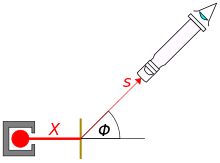s = the number of alpha particles falling on unit area at an angle of deflection Φ
r = distance from point of incidence of α rays on scattering material
X = total number of particles falling on the scattering material
n = number of atoms in a unit volume of the material
t = thickness of the foil
Qn = positive charge of the atomic nucleus
Qα = positive charge of the alpha particles
m = mass of an alpha particle
v = velocity of the alpha particle

### The 1913 experiment

In a 1913 paper, The Laws of Deflexion of α Particles through Large Angles, Geiger and Marsden describe a series of experiments by which they sought to experimentally verify the above equation that Rutherford developed. Rutherford's equation predicted that the number of scintillations per minute s that will be observed at a given angle Φ should be proportional to:

1. csc4Φ/2
2. thickness of foil t
3. magnitude of central charge Qn
4. 1/(mv2)2

Their 1913 paper describes four experiments by which they proved each of these four relationships.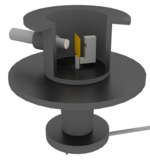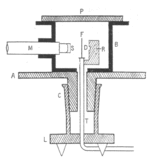This apparatus was described in a 1913 paper by Geiger and Marsden. It was designed to accurately measure the scattering pattern of the alpha particles produced by the metal foil (F). The microscope (M) and screen (S) were affixed to a rotating cylinder and could be moved a full circle around the foil so that they could count scintillations from every angle.

To test how the scattering varied with the angle of deflection (i.e. if s ∝ csc4Φ/2) Geiger and Marsden built an apparatus that consisted of a hollow metal cylinder mounted on a turntable. Inside the cylinder was a metal foil (F) and a radiation source containing radon (R), mounted on a detached column (T) which allowed the cylinder to rotate independently. The column was also a tube by which air was pumped out of the cylinder. A microscope (M) with its objective lens covered by a fluorescent zinc sulfide screen (S) penetrated the wall of the cylinder and pointed at the metal foil. By turning the table, the microscope could be moved a full circle around the foil, allowing Geiger to observe and count alpha particles deflected by up to 150°. Correcting for experimental error, Geiger and Marsden found that the number of alpha particles that are deflected by a given angle Φ is indeed proportional to csc4Φ/2.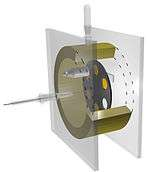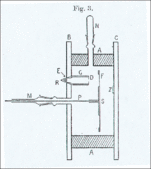This apparatus was used to measure how the alpha particle scattering pattern varied in relation to the thickness of the foil, the atomic weight of the material, and the velocity of the alpha particles. The rotating disc in the center had six holes which could be covered with foil.

Geiger and Marsden then tested how the scattering varied with the thickness of the foil (i.e. if s ∝ t). They constructed a disc (S) with six holes drilled in it. The holes were covered with metal foil (F) of varying thickness, or none for control. This disc was then sealed in a brass ring (A) between two glass plates (B and C). The disc could be rotated by means of a rod (P) to bring each window in front of the alpha particle source (R). On the rear glass pane was a zinc sulfide screen (Z). Geiger and Marsden found that the number of scintillations that appeared on the zinc sulfide screen was indeed proportional to the thickness as long as said thickness was small.

Geiger and Marsden reused the above apparatus to measure how the scattering pattern varied with the square of the nuclear charge (i.e. if s ∝ Qn2). Geiger and Marsden didn't know what the positive charge of the nucleus of their metals were (they had only just discovered the nucleus existed at all), but they assumed it was proportional to the atomic weight, so they tested whether the scattering was proportional to the atomic weight squared. Geiger and Marsden covered the holes of the disc with foils of gold, tin, silver, copper, and aluminum. They measured each foil's stopping power by equating it to an equivalent thickness of air. They counted the number of scintillations per minute that each foil produced on the screen. They divided the number of scintillations per minute by the respective foil's air equivalent, then divided again by the square root of the atomic weight (Geiger and Marsden knew that for foils of equal stopping power, the number of atoms per unit area is proportional to the square root of the atomic weight). Thus, for each metal, Geiger and Marsden obtained the number of scintillations that a fixed number of atoms produce. For each metal, they then divided this number by the square of the atomic weight, and found that the ratios were more or less the same. Thus they proved that s ∝ Qn2.

Finally, Geiger and Marsden tested how the scattering varied with the velocity of the alpha particles (i.e. if s ∝ 1/v4). Using the same apparatus again, they slowed the alpha particles by placing extra sheets of mica in front of the alpha particle source. They found that, within the range of experimental error, that the number of scinitillations was indeed proportional to 1/v4.

### Rutherford determines the nucleus is positively charged

In his 1911 paper (see above), Rutherford assumed that the central charge of the atom was positive, but a negative charge would have fitted his scattering model just as well. In a 1913 paper, Rutherford declared that the "nucleus" (as he now called it) was indeed positively charged, based on the result of experiments exploring the scattering of alpha particles in various gases.

In 1917, Rutherford and his assistant William Kay began exploring the passage of alpha particles through gases such as hydrogen and nitrogen. In an experiment where they shot a beam of alpha particles through hydrogen, the alpha particles knocked the hydrogen nuclei forwards in the direction of the beam, not backwards. In an experiment where they shot alpha particles through nitrogen, he discovered that the alpha particles knocked hydrogen nuclei (i.e. protons) out of the nitrogen nuclei.

## Legacy

When Geiger reported to Rutherford that he had spotted alpha particles being strongly deflected, Rutherford was astounded. In a lecture Rutherford delivered at Cambridge University, he said:

It was quite the most incredible event that has ever happened to me in my life. It was almost as incredible as if you fired a 15-inch shell at a piece of tissue paper and it came back and hit you. On consideration, I realized that this scattering backward must be the result of a single collision, and when I made calculations I saw that it was impossible to get anything of that order of magnitude unless you took a system in which the greater part of the mass of the atom was concentrated in a minute nucleus. It was then that I had the idea of an atom with a minute massive centre, carrying a charge.
Ernest Rutherford

Accolades soon flooded in. Hantaro Nagaoka, who had once proposed a Saturnian model of the atom, wrote to Rutherford from Tokyo in 1911: "Congratulations on the simpleness of the apparatus you employ and the brilliant results you obtained". The conclusions of these experiments revealed how all matter on Earth is structured and thus affected every scientific and engineering discipline, making it one of the most pivotal scientific discoveries of all time. The astronomer Arthur Eddington called Rutherford's discovery the most important scientific achievement since Democritus proposed the atom ages earlier.

Like most scientific models, Rutherford's atomic model was neither perfect nor complete. According to classical Newtonian physics, it was in fact impossible. Accelerating charged particles radiate electromagnetic waves, so an electron orbiting an atomic nucleus in theory would spiral into the nucleus as it loses energy. To fix this problem, scientists had to incorporate quantum mechanics into Rutherford's model.